# Very Short Answer Type Questions- Areas Related to Circles Class 10 Notes | EduRev

## Class 10 : Very Short Answer Type Questions- Areas Related to Circles Class 10 Notes | EduRev

The document Very Short Answer Type Questions- Areas Related to Circles Class 10 Notes | EduRev is a part of the Class 10 Course Class 10 Mathematics by VP Classes.
All you need of Class 10 at this link: Class 10

Q1. PQRS is a diameter of a circle of radius 6 cm. The equal lengths PQ, QR and RS are drawn on PQ and QS as diameters, as shown in figure. Find the perimeter of the shaded region.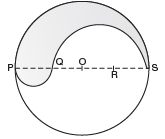Sol. Diameter PS = 12 cm      [∵ Radius OS = 6 cm]

Since PQ, QR and RS are three equal parts of diameter,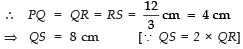Now, the total required perimeter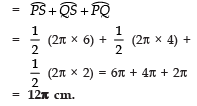Q2. A sheet of paper is in the form of a rectangle ABCD in which AB = 40 cm, and BC = 28 cm. A semi-circlular portion with BC as diameter is cut off. Find the area of the remaining paper.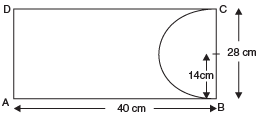Sol. Length of the paper = 40 cm, width of the paper = 28 cm.
∴ Area of the rectangle =length × breadth = 40 × 28 cm2 = 1120 cm2
Again, diameter of semi-circle = 28 cm.
⇒ Radius of the semi-circle = 28/2 = 14 cm.

∴ Area of the semi-circle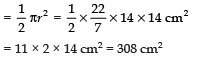∴ Area of the remaining paper = 1120 cm2 − 308 cm2 = 812 cm2.

Q3. Find the area of the shaded region in the figure, if ABCD is a square of side 14 cm and APD and BPC are semi-circles.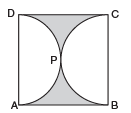Sol. Side of the square = 14 cm
∴ Area of the square = 14 × 14 cm2 = 196 cm2

Also, diameter of each semi-circle = side of the square = 14 cm.
⇒ Radius =  14/2 = = 7 cm.
Area of 1 semi-circle = 1/2 πr2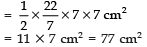⇒ Area of both semi-circles = 2 × 77 cm2
= 154 cm2.
Now, the area of the shaded region = [Area of the square ABCD] − [Area of both the semi-circles]
= (196 − 154) cm2 = 42 cm2.

Q4. Find the perimeter of the shaded region, if ABCD is a square of side 21 cm and APB and CPD are semi-circles. (use π = 22/7)

Sol. Here diameter = 21 cm ⇒  r = 21/2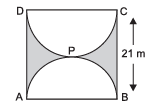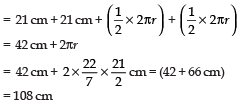Thus the required perimeter of shaded region is 108 cm.

Q5. A park is in the form of a rectangle 120 m long and 100 m wide. At the centre, there is a circular lawn of radius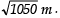Find the area of the park excluding the lawn.

[Take π = 22/7]

Sol. Length of the park l = 120 m
Breadth of the park b = 100 m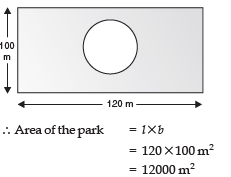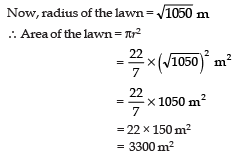∴ Area of the park excluding the central park

= 12000 − 3300 m2
= 8700 m2.

Q6. In the given figure, OAPB is a sector of a circle of radius 3.5 cm with the centre at O and ∠AOB = 120°. Find the length of OAPBO.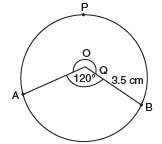Sol. Here, the major sector angle is given by θ = 360° − 120° = 240°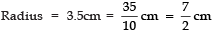∴ Circumference of the sector APB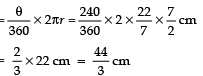∴ Perimeter of OAPBO = [Circumference of sector AOB] + OA + OB]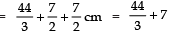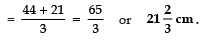Q7. Find the area of the shaded region of the following figure, if the diameter of the circle with centre O is 28 cm and AQ = 1/4 AB.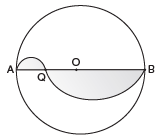Sol. We have AB = 28 cm

∴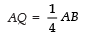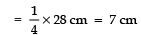⇒ BQ = 28 − 7 = 21 cm
∴ Area of the semi-circle having diameter as 21 cm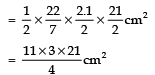∴ Also area of the semi-circle having diameter as 7 cm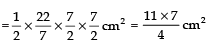Thus the area of the shaded region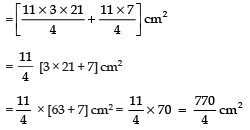= 192.5 cm2.

Q8. PQRS is a square land of side 28 m. Two semi-circular grass covered postions are to be made on two of its opposite sides as shown in the figure. How much area will be left uncovered? [Take π = 22/7]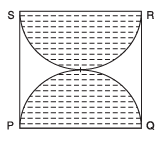Sol. Side of the square = 28 m
∴ Area of the square PQRS = 28 × 28 m2
Diameter of a semi-circle = 28 m

⇒ Radius of a semi-circle = 28/2 = 14m

∴ Area of 1 semi-circle = 1/2πr2  =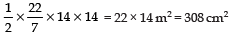⇒ Area of both the semi-circles = 2 × 308 m2 = 616 m2

∴ Area of the square left uncovered = (28 × 28) − 616 m2 = 784 − 616 m2 = 168 m2.

Q9. Find the area of a square inscribed in a circle of radius 10 cm.

Sol. Let ABCD be the square such that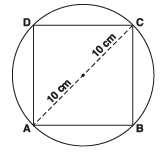AB = BC = 10 cm
∴ AC2 = AB2 + BC2
AB2 + BC2 = (10 × 2)
⇒ x2 + x2 = (20)2
[Let AB = BC = x]
⇒ 2x2 = 400

⇒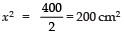∴ Area of the square = 200 cm2.

Q10. In the given figure, O is the centre of a circular arc and AOB is a straight line. Find the perimeter of the shaded region.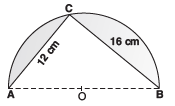Sol. O is the centre of the circle.
∴ AB is its diameter.
In right Δ ABC,
AC2 + BC2 = AB2
⇒ 122 + 162 = AB2
⇒ 144 + 256 = AB2 ⇒ AB2 = 400

⇒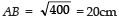∴ Circumference of semi-circle ACB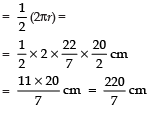∴ Perimeter of the shaded region = 22/7 cm + 12 cm + 16 cm
= 31.43 cm + 12cm + 16cm
= 59.43 cm

Offer running on EduRev: Apply code STAYHOME200 to get INR 200 off on our premium plan EduRev Infinity!

132 docs

,

,

,

,

,

,

,

,

,

,

,

,

,

,

,

,

,

,

,

,

,

;